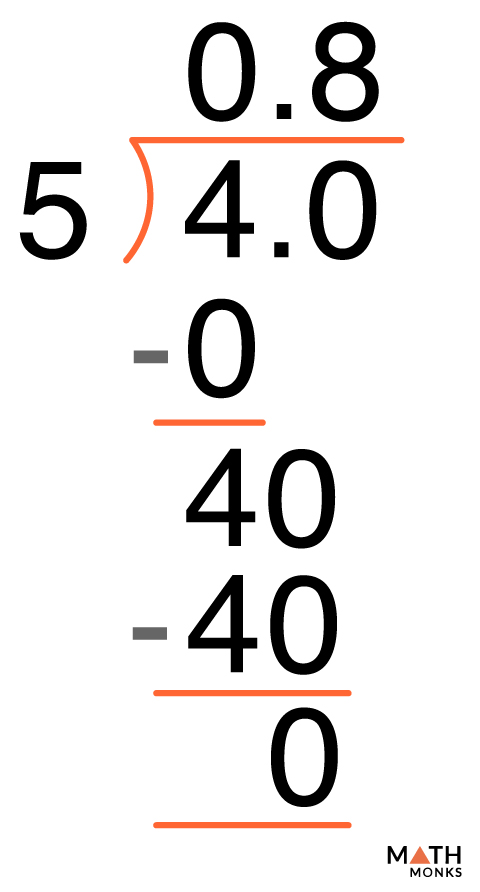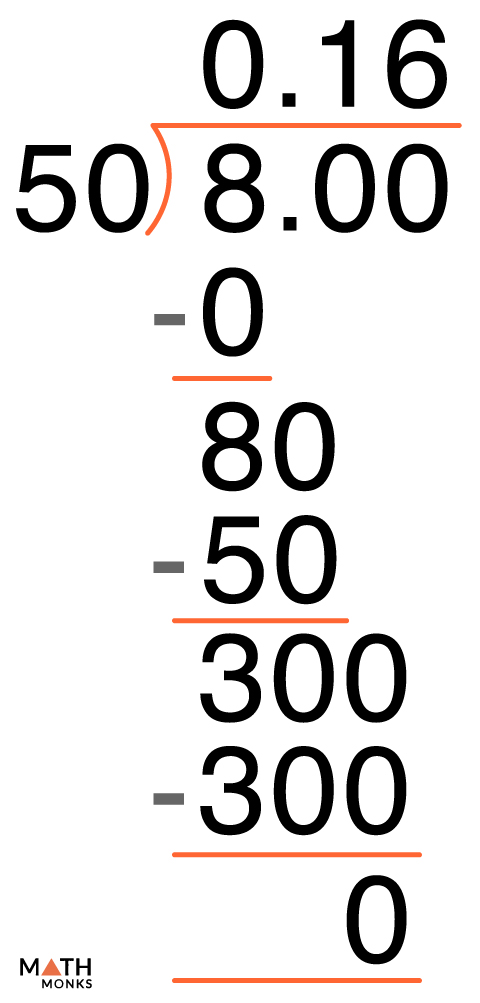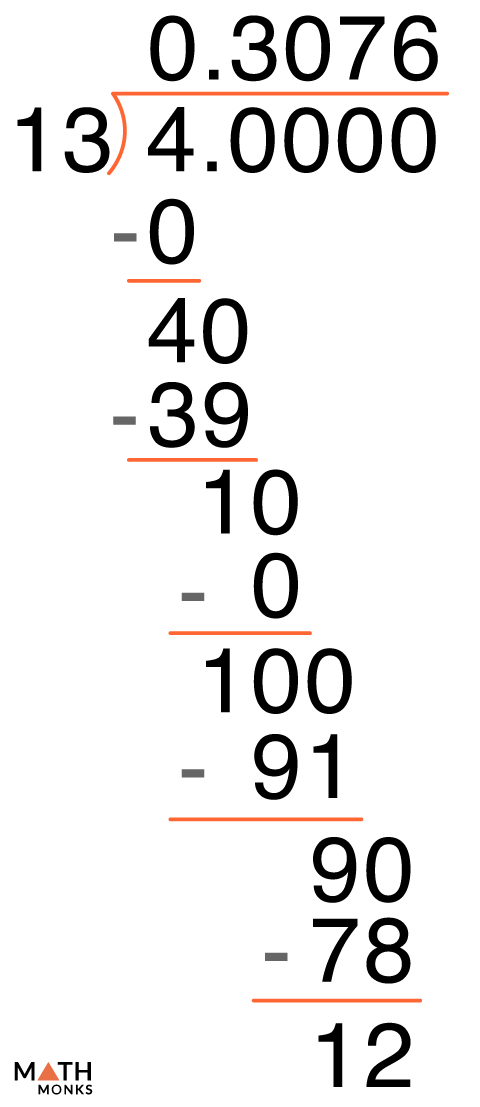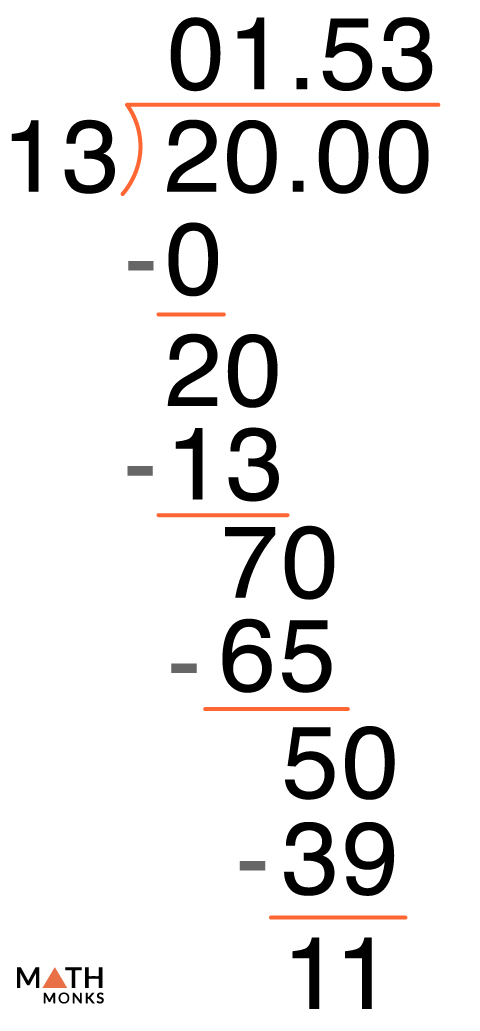# Fraction to Decimal

Converting fractions to decimals is to write a decimal as a fraction in the simplest form. In other words, we change a decimal to p/q form, where q is non-zero. Here we will discuss the ways to convert fractions to decimals.

## Fraction to Decimal Chart

We can use a fraction to decimal conversion chart in order to memorize some commonly used fractions. A printable conversion chart is given below for your use.

## How to Convert Fraction to Decimal

### With Calculator

The easiest way to turn any fraction to decimal is to use an electronic calculator. We type the fraction in p/q form and find the result on the screen of the calculator. For example, if we need to find the decimal for ${\dfrac{16}{19}}$, we will press the buttons in the following sequence :

1. 16
2. /
3. 19
4. =

We get the result ‘0.8421…’ The result is a non-terminating decimal as the remainders are never zero.

### With Long Division

As we have learned the basic steps for dividing decimals, we will apply the same while converting a fraction into a decimal.

Let us solve some examples.

Change the fraction 4/5 to a decimal.Solution:

∴ Fraction 4/5 to decimal is 0.8

Change the fraction 8/50 to a decimal.Solution:

∴ Fraction 8/50 to decimal is 0.16

Change the fraction 4/13 to a decimal.Solution:

Change the fraction 13/20 to a decimal.Solution:

### Alternative Method

We can apply another way to change a decimal to fraction. The steps are:

1. Find a number to multiply with the denominator to change it to 10, or 100, or 1000, or a 1 followed by zero(s). (if its 5, we multiply it with 2 to make it 10, if its 25, we multiply with 4 to make it 100). Apply it on numbers that can be easily changed to multiples of 10.
2. Multiply both the numerator and denominator with that number.
3. Then write the top number, and put the decimal point in the correct place (one place from the digit to the extreme right for every zero in the denominator. For 653/10, it will be 65.3, and for 653/100, it will be 6.53)]

For example, ${\dfrac{3}{4}}$ ${=\dfrac{3\times 25}{4\times 25}=\dfrac{75}{100}=7.5}$

Express ${\dfrac{3}{16}}$ as decimal.

Solution:

We try to change 16 to 100. So we divide 100 by 16 and get 6.25
${\therefore \dfrac{3\times .6\cdot 25}{16\times 6\cdot 25}}$, multiplying both top and bottom with 6.25,
= ${\dfrac{18.75}{100}}$
= 0.1875

Convert ${\dfrac{2}{3}}$

Solution:

There is no way we can change 3 to 10. So we try to make it close to 10 or 100. But, we can calculate the approximate decimal. So we will change the bottom number close to 1000.
${\dfrac{2\times 333}{3\times 333}=\dfrac{666}{999}}$
We will assume 999 as 1,000. So we will put the decimal place accordingly.
${\cong \dfrac{666}{1000}}$
= 0.666